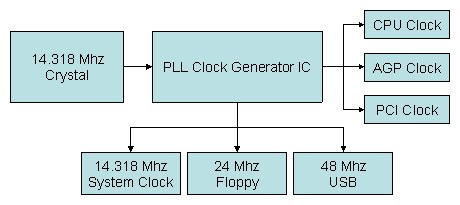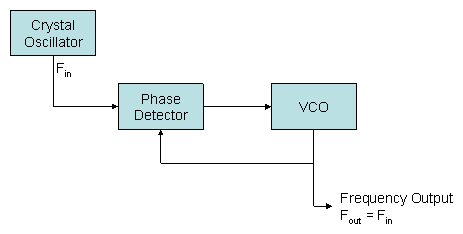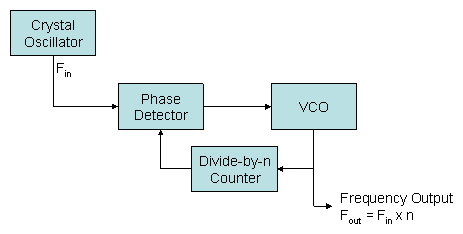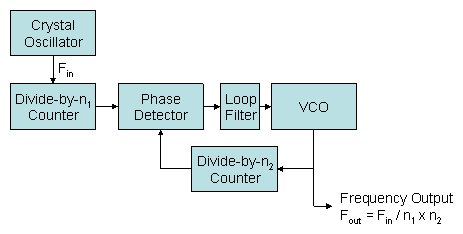# What is a PLL?

Author: W1zzard
Date: 2004-05-24 07:09:03

A phase-locked loop (PLL) is an electronic circuit with a voltage- or current-driven oscillator that is constantly adjusted to match in phase (and thus locked on) the frequency of an input signal. PLLs are used for frequency control. They can be configured as frequency multipliers, dividers, demodulators, tracking generators or clock recovery circuits.

On a computer motherboard a PLL is used to generate several frequencies that are required for proper operation:Let's try to build our own PLL: What we want is a stable frequency that we can change on the fly.
A crystal oscillator would give us a stable frequency- but it's fixed and using several oscillators would be a waste of resources.
If we use a VCO (Voltage Controlled Oscillator) we get a freely changeable frequency but the frequency would change even at the slightest variation of voltage. If there was a way to combine the stability of the crystal oscillator with the flexibility of the VCO we would have the perfect solution for our problem.

What if we add a phase detector? A phase detector is a device that takes two signals. If both have the same phase and frequency the output is zero. If the signals are out-of-phase the output will be a DC voltage that is proportional to the phase difference between the two inputs.What does this do? The crystal generates a frequency of 10 MHz in our example. This frequency gets fed to the PD. The VCO output is zero right now so the PD output is high because the frequencies differ a lot. The PD output gets fed into the VCO and generates a frequency. This goes back to the PD and so on - eventually the VCO will lock onto the frequency of the crystal. And the output of the VCO will be 10 MHz. This is a basic PLL circuit.
Now you ask where's the flexibility? What we have right now is equivalent to a crystal oscillator!

Let's see.. We want an output of 20 Mhz for this example. What we do now is add a divide-by-two counter between the VCO output and the PD. This 'tricks' the PD into thinking our output frequency is only the half of what it really is- so it starts regulating the voltage to the VCO. Voila 20 MHz. If we used a programmable divider here we could change frequencies on the fly.Now we can multiply frequencies by integer values. But how can we multiply our frequency by fractional values or divide it?
For example we want 10 Mhz x 3.5 for an output of 35 Mhz. This is done by adding another pre-divider [whats the correct term here?] between the crystal and the PD. In our example we could use a pre-divider of 2 and a divider of 7. Our output would be 10 Mhz / 2 * 7 = 35 MHz.

This is our PLL in all its goodness:The added loop filter is not required for general understanding. It's here for completeness. The loop filter is designed to match the characteristics required by the application of the PLL. For example, it determines how fast the signal frequency can change and still maintain lock. This is the maximum slewing rate. The narrower the loop filter bandwidth the smaller the achievable phase error. This comes at the expense of slower response and reduced capture range.